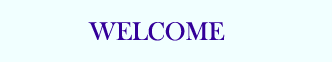TUTORIALS

C Quiz
C Exam

# Functions with Variable Argument Lists in C using va_list

Perhaps you would like to have a function that will accept any number of values and then return the average. You don't know how many arguments will be passed in to the function. One way you could make the function would be to accept a pointer to an array. Another way would be to write a function that can take any number of arguments. So you could write avg(4, 12.2, 23.3, 33.3, 12.1); or you could write avg(2, 2.3, 34.4); The advantage of this approach is that it's much easier to change the code if you want to change the number of arguments. Indeed, some library functions can accept a variable list of arguments (such as printf--I bet you've been wondering how that works!).

Whenever a function is declared to have an indeterminate number of arguments, in place of the last argument you should place an ellipsis (which looks like '...'), so, int a_function ( int x, ... ); would tell the compiler the function should accept however many arguments that the programmer uses, as long as it is equal to at least one, the one being the first, x.

We'll need to use some macros (which work much like functions, and you can treat them as such) from the stdarg.h header file to extract the values stored in the variable argument list--va_start, which initializes the list, va_arg, which returns the next argument in the list, and va_end, which cleans up the variable argument list.

To use these functions, we need a variable capable of storing a variable-length argument list--this variable will be of type va_list. va_list is like any other type. For example, the following code declares a list that can be used to store a variable number of arguments.
```va_list a_list;
```
va_start is a macro which accepts two arguments, a va_list and the name of the variable that directly precedes the ellipsis ("..."). So in the function a_function, to initialize a_list with va_start, you would write va_start ( a_list, x );
```int a_function ( int x, ... )
{
va_list a_list;
va_start( a_list, x );
}
```
va_arg takes a va_list and a variable type, and returns the next argument in the list in the form of whatever variable type it is told. It then moves down the list to the next argument. For example, va_arg ( a_list, double ) will return the next argument, assuming it exists, in the form of a double. The next time it is called, it will return the argument following the last returned number, if one exists. Note that you need to know the type of each argument--that's part of why printf requires a format string! Once you're done, use va_end to clean up the list: va_end( a_list );

To show how each of the parts works, take an example function:
```#include <stdarg.h>
#include <stdio.h>

/* this function will take the number of values to average
followed by all of the numbers to average */
double average ( int num, ... )
{
va_list arguments;
double sum = 0;

/* Initializing arguments to store all values after num */
va_start ( arguments, num );
/* Sum all the inputs; we still rely on the function caller to tell us how
* many there are */
for ( int x = 0; x < num; x++ )
{
sum += va_arg ( arguments, double );
}
va_end ( arguments );                  // Cleans up the list

return sum / num;
}

int main()
{
/* this computes the average of 13.2, 22.3 and 4.5 (3 indicates the number of values to average) */
printf( "%f\n", average ( 3, 12.2, 22.3, 4.5 ) );
/* here it computes the average of the 5 values 3.3, 2.2, 1.1, 5.5 and 3.3
printf( "%f\n", average ( 5, 3.3, 2.2, 1.1, 5.5, 3.3 ) );
}
```
It isn't necessarily a good idea to use a variable argument list at all times; the potential exists for assuming a value is of one type, while it is in fact another, such as a null pointer being assumed to be an integer. Consequently, variable argument lists should be used sparingly.